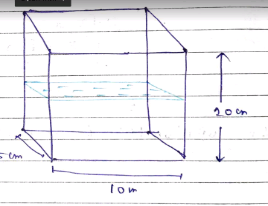A rectangular tank has a base that is 10 cm by 5 cm and a height of 20 cm. If the tank is half full of water, by how many centimetres will the water level rise if 325 cm3 are poured in the tank?

# A rectangular tank has a base that is 10 cm by 5 cm and a height of 20 cm. If the tank is half full of water, by how many centimetres will the water level rise if 325 cm3 are poured in the tank?

1. A
6.5 cm
2. B
7 cm
3. C
8 cm
4. D
10 cm

Fill Out the Form for Expert Academic Guidance!l

+91

Live ClassesBooksTest SeriesSelf Learning

Verify OTP Code (required)

### Solution:

The volume of the half -filled tank
=l$×$b$×$($\frac{n}{2}$)
=10$×$5$×\frac{20}{5}$
=500 cm3Now water is added to the tank , and the volume of water increase
V1 = v+325
= 500+325
= 825 cm3
V1 = l$×$b$×$h
825  = 10$×$5$×$x
X = 16.5 cm
Now increase in level = 16.5 cm -10cm
= 6.5 cm.

## Related content

 Area of Square Area of Isosceles Triangle Pythagoras Theorem Triangle Formula Perimeter of Triangle Formula Area Formulae Volume of Cone Formula Matrices and Determinants_mathematics Critical Points Solved Examples Type of relations_mathematics+91

Live ClassesBooksTest SeriesSelf Learning

Verify OTP Code (required)